# Theory of Wind Turbine and Betz Coefficient

For determining power extracted from wind by wind turbine we have to assume an air duct as shown in the figure. It is also assumed that the velocity of the wind at the inlet of the duct is V1 and velocity of air at the outlet of the duct is V2. Say, mass m of the air is passed through this imaginary duct per second.
Now due to this mass the kinetic energy of wind at the inlet of the duct is,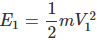Similarly, due to this mass the kinetic energy of wind at the outlet of the duct is,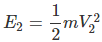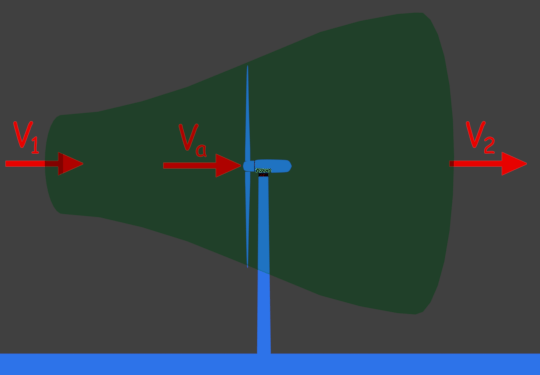Hence, the kinetic energy of wind changed, during the flow of this quantity of air from the inlet to the outlet of the imaginary duct is,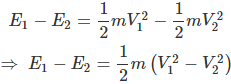As we already said that, mass m of the air is passed through this imaginary duct in one second. Hence the power extracted from the wind is the same as the kinetic energy changed during the flow of mass m of the air from the inlet to the outlet of the duct.

We define power as the change of energy per second. Hence, this extracted power can be written as,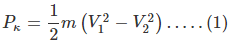As mass m of the air passes in one second, we refer the quantity m as the mass flow rate of the wind. If we think of that carefully, we can easily understand that mass flow rate will be the same at the inlet, at the outlet and as well as at every cross-section of the air duct. Since, whatever quantity of air is entering the duct, the same is coming out from the outlet.
If Va, A and ρ are the velocity of the air, the cross-sectional area of the duct and density of air at the turbine blades respectively, then the mass flow rate of the wind can be represented as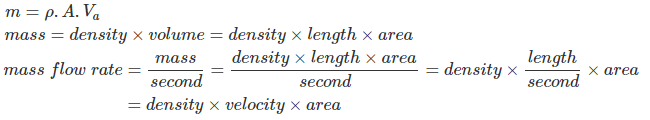Now, replacing m by ρVaA in equation (1), we get,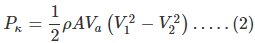Now, as the turbine is assumed to be placed at the middle of the duct, the wind velocity at turbine blades can be considered as average velocity of inlet and outlet velocities.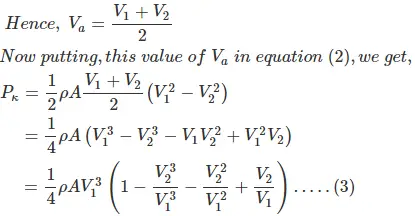To obtain maximum power from wind, we have to differentiate equation (3) in respect of V2 and equate it to zero. That is,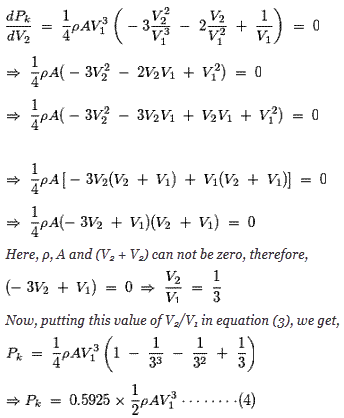## Betz Coefficient

From, the above equation it is found that the theoretical maximum power extracted from the wind is in the fraction of 0.5925 of its total kinetic power. This fraction is known as the Betz Coefficient. This calculated power is according to theory of wind turbine but actual mechanical power received by the generator is lesser than that and it is due to losses for friction rotor bearing and inefficiencies of aerodynamic design of the turbine.

From equation (4) it is clear that the extracted power is

1. Directly proportional to air density ρ. As air density increases, the power of the turbine increases.
2. Directly proportional to the swept area of the turbine blades. If the length of the blade increases, the radius of the swept area increases accordingly, so turbine power increases.
3. Turbine power also varies with velocity3 of the wind. That indicates if the velocity of wind doubles and the turbine power will increase to eight folds.Want To Learn Faster? 🎓
Get electrical articles delivered to your inbox every week.
No credit card required—it’s 100% free.